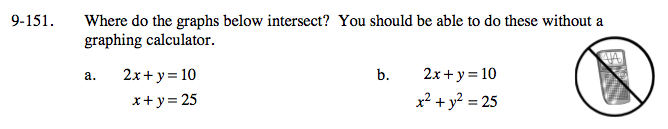### Home > A2C > Chapter Ch9 > Lesson 9.3.2 > Problem9-151

9-151.
1. Where do the graphs below intersect? You should be able to do these without a graphing calculator. Homework Help ✎1. 2x + y = 10
x + y = 25

2. 2x + y = 10
x2 + y2 = 25Multiply the second equation by −1 and add the two equations together.

2x + y = 10
xy = −25

x = −15

Use the x-value to calculate the y.

y − 15 = 25

y = 40

(−15, 40)

Solve the first equation for y and substitute it into the second.